2022: SklogWiki celebrates 15 years on-line

Semi-grand ensembles

(Redirected from Semigrand ensembles)

Semi-grand ensembles are used in Monte Carlo simulation of mixtures. In these ensembles the total number of molecules is fixed, but the composition can change.

Canonical ensemble: fixed volume, temperature and number(s) of molecules

We shall consider a system consisting of c components;. In the canonical ensemble, the differential equation energy for the Helmholtz energy function can be written as: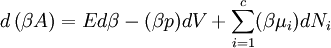$d \left( \beta A \right) = E d \beta - (\beta p) d V + \sum_{i=1}^c (\beta \mu_i) d N_i$,

where:

•$A$ is the Helmholtz energy function
•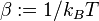$\beta := 1/k_B T$
•$k_B$ is the Boltzmann constant
•$T$ is the absolute temperature
•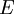$E$ is the internal energy
•$p$ is the pressure
•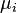$\mu_i$ is the chemical potential of the species$i$
•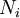$N_i$ is the number of molecules of the species$i$

Semi-grand ensemble at fixed volume and temperature

Consider now that we wish to consider a system with fixed total number of particles,$N$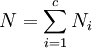$\left. N = \sum_{i=1}^c N_i \right.$;

but the composition can change, from thermodynamic considerations one can apply a Legendre transform [HAVE TO CHECK ACCURACY] to the differential equation written above in terms of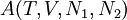$A (T,V,N_1,N_2)$.

• Consider the variable change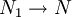$N_1 \rightarrow N$ i.e.: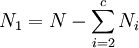$\left. N_1 = N- \sum_{i=2}^c N_i \right.$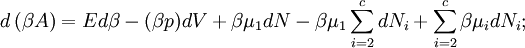$d \left( \beta A \right) = E d \beta - (\beta p) d V + \beta \mu_1 d N - \beta \mu_1 \sum_{i=2}^c d N_i + \sum_{i=2}^c \beta \mu_i d N_i;$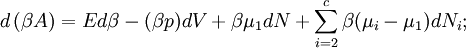$d \left( \beta A \right) = E d \beta - (\beta p) d V + \beta \mu_1 d N + \sum_{i=2}^c \beta (\mu_i-\mu_1) d N_i;$

or,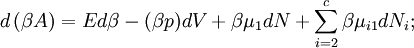$d \left( \beta A \right) = E d \beta - (\beta p) d V + \beta \mu_1 d N + \sum_{i=2}^c \beta \mu_{i1} d N_i;$

where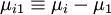$\left. \mu_{i1} \equiv \mu_i - \mu_1 \right.$.

• Now considering the thermodynamic potential: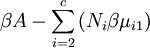$\beta A - \sum_{i=2}^c \left( N_i \beta \mu_{i1} \right)$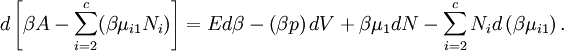$d \left[ \beta A - \sum_{i=2}^c ( \beta \mu_{i1} N_i ) \right] = E d \beta - \left( \beta p \right) d V + \beta \mu_{1} d N - \sum_{i=2}^c N_i d \left( \beta \mu_{i1} \right).$

Fixed pressure and temperature

In the isothermal-isobaric ensemble: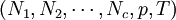$(N_1,N_2, \cdots, N_c, p, T)$ one can write: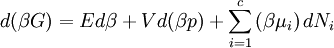$d (\beta G) = E d \beta + V d (\beta p) + \sum_{i=1}^c \left( \beta \mu_i \right) d N_i$

where:

•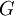$G$ is the Gibbs energy function

Fixed pressure and temperature: Semi-grand ensemble

Following the procedure described above one can write: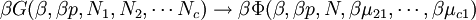$\beta G (\beta,\beta p, N_1, N_2, \cdots N_c ) \rightarrow \beta \Phi (\beta, \beta p, N, \beta \mu_{21}, \cdots, \beta \mu_{c1} )$,

where the new thermodynamic potential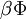$\beta \Phi$ is given by: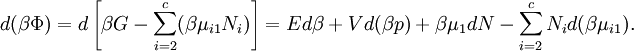$d (\beta \Phi) = d \left[ \beta G - \sum_{i=2}^c (\beta \mu_{i1} N_i ) \right] = E d \beta + V d (\beta p) + \beta \mu_1 d N - \sum_{i=2}^c N_i d (\beta \mu_{i1} ).$

Fixed pressure and temperature: Semi-grand ensemble: partition function

In the fixed composition ensemble one has: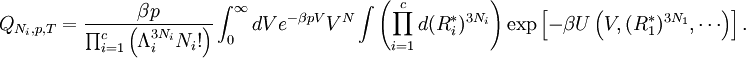$Q_{N_i,p,T} = \frac{ \beta p }{\prod_{i=1}^c \left( \Lambda_i^{3N_i} N_i! \right) } \int_{0}^{\infty} dV e^{-\beta p V } V^N \int \left( \prod_{i=1}^c d (R_i^*)^{3N_i} \right) \exp \left[ - \beta U \left( V, (R_1^*)^{3N_1} , \cdots \right) \right].$